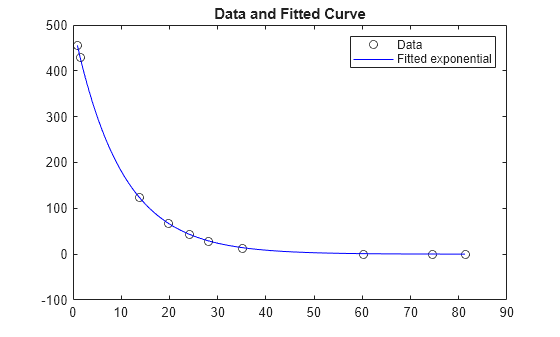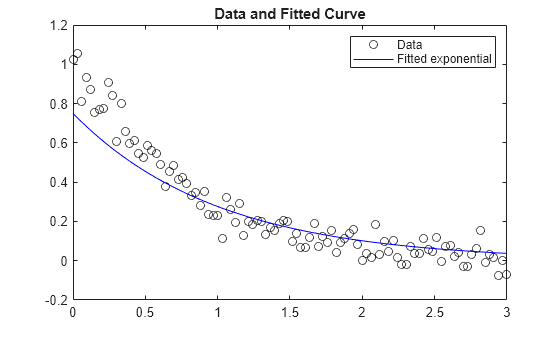# lsqcurvefit

## 语法

``x = lsqcurvefit(fun,x0,xdata,ydata)``
``x = lsqcurvefit(fun,x0,xdata,ydata,lb,ub)``
``x = lsqcurvefit(fun,x0,xdata,ydata,lb,ub,options)``
``x = lsqcurvefit(problem)``
``[x,resnorm] = lsqcurvefit(___)``
``[x,resnorm,residual,exitflag,output] = lsqcurvefit(___)``
``[x,resnorm,residual,exitflag,output,lambda,jacobian] = lsqcurvefit(___)``

## 说明

`$\underset{x}{\mathrm{min}}{‖F\left(x,xdata\right)-ydata‖}_{2}^{2}=\underset{x}{\mathrm{min}}\sum _{i}{\left(F\left(x,xdat{a}_{i}\right)-ydat{a}_{i}\right)}^{2},$`

（可选）x 的分量可以有下界和上界，即 lb 和 ub。参数 x、lb 和 ub 可以是向量或矩阵；请参阅矩阵参数

`lsqcurvefit` 函数使用与 `lsqnonlin` 相同的算法。`lsqcurvefit` 只是为数据拟合问题提供一个方便的接口。

`lsqcurvefit` 要求基于用户定义的函数来计算向量值函数

`$F\left(x,xdata\right)=\left[\begin{array}{c}F\left(x,xdata\left(1\right)\right)\\ F\left(x,xdata\left(2\right)\right)\\ ⋮\\ F\left(x,xdata\left(k\right)\right)\end{array}\right].$`

，而不是计算平方和

````x = lsqcurvefit(fun,x0,xdata,ydata)` 从 `x0` 开始，求取合适的系数 `x`，使非线性函数 `fun(x,xdata)` 对数据 `ydata` 的拟合最佳（基于最小二乘指标）。`ydata` 必须与 `fun` 返回的向量（或矩阵）`F` 大小相同。 注意传递额外参数说明如何在必要时为向量函数 `fun(x)` 传递额外参数。 ```

````x = lsqcurvefit(fun,x0,xdata,ydata,lb,ub)` 对 `x` 中的设计变量定义一组下界和上界，使解始终在 `lb `≤` x `≤` ub` 范围内。您可以通过指定 `lb(i) = ub(i)` 来修复解分量 `x(i)`。 注意如果问题的指定输入边界不一致，则输出 `x` 为 `x0`，输出 `resnorm` 和 `residual` 为 `[]`。违反边界 `lb ≤ x ≤ ub` 的 `x0` 的分量将重置为位于由边界定义的框内。遵守边界的分量不会更改。 ```

``x = lsqcurvefit(fun,x0,xdata,ydata,lb,ub,options)` 使用 `options` 所指定的优化选项执行最小化。使用 `optimoptions` 可设置这些选项。如果不存在边界，则为 `lb` 和 `ub` 传递空矩阵。`
``x = lsqcurvefit(problem)` 求 `problem` 的最小值，它是 `problem` 中所述的一个结构体。`
`对于任何输入参数，`[x,resnorm] = lsqcurvefit(___)` 返回在 `x` 处的残差的 2-范数平方值：`sum((fun(x,xdata)-ydata).^2)`。`

``[x,resnorm,residual,exitflag,output] = lsqcurvefit(___)` 还返回在解 `x` 处的残差 `fun(x,xdata)-ydata` 的值、描述退出条件的值 `exitflag`，以及包含优化过程信息的结构体 `output`。`
``[x,resnorm,residual,exitflag,output,lambda,jacobian] = lsqcurvefit(___)` 还返回结构体 `lambda`（其字段包含在解 `x` 处的拉格朗日乘数），以及 `fun` 在解 `x` 处的 Jacobian 矩阵。`

## 示例

`$\text{ydata}=x\left(1\right)\mathrm{exp}\left(x\left(2\right)\text{xdata}\right).$`

```xdata = ... [0.9 1.5 13.8 19.8 24.1 28.2 35.2 60.3 74.6 81.3]; ydata = ... [455.2 428.6 124.1 67.3 43.2 28.1 13.1 -0.4 -1.3 -1.5];```

`fun = @(x,xdata)x(1)*exp(x(2)*xdata);`

`x0 = [100,-1]` 为起点拟合模型。

```x0 = [100,-1]; x = lsqcurvefit(fun,x0,xdata,ydata)```
```Local minimum possible. lsqcurvefit stopped because the final change in the sum of squares relative to its initial value is less than the value of the function tolerance. ```
```x = 1×2 498.8309 -0.1013 ```

```times = linspace(xdata(1),xdata(end)); plot(xdata,ydata,'ko',times,fun(x,times),'b-') legend('Data','Fitted exponential') title('Data and Fitted Curve')````$y=\mathrm{exp}\left(-1.3t\right)+\epsilon ,$`

```rng default % for reproducibility xdata = linspace(0,3); ydata = exp(-1.3*xdata) + 0.05*randn(size(xdata));```

`$0\le x\left(1\right)\le 3/4$`

`$-2\le x\left(2\right)\le -1.$`

```lb = [0,-2]; ub = [3/4,-1];```

`fun = @(x,xdata)x(1)*exp(x(2)*xdata);`

`x0 = [1/2,-2];`

`x = lsqcurvefit(fun,x0,xdata,ydata,lb,ub)`
```Local minimum found. Optimization completed because the size of the gradient is less than the value of the optimality tolerance. ```
```x = 1×2 0.7500 -1.0000 ```

```plot(xdata,ydata,'ko',xdata,fun(x,xdata),'b-') legend('Data','Fitted exponential') title('Data and Fitted Curve')````$\text{ydata}=x\left(1\right)\mathrm{exp}\left(x\left(2\right)\text{xdata}\right).$`

```xdata = ... [0.9 1.5 13.8 19.8 24.1 28.2 35.2 60.3 74.6 81.3]; ydata = ... [455.2 428.6 124.1 67.3 43.2 28.1 13.1 -0.4 -1.3 -1.5];```

`fun = @(x,xdata)x(1)*exp(x(2)*xdata);`

`x0 = [100,-1]` 为起点拟合模型。

```x0 = [100,-1]; x = lsqcurvefit(fun,x0,xdata,ydata)```
```Local minimum possible. lsqcurvefit stopped because the final change in the sum of squares relative to its initial value is less than the value of the function tolerance. ```
```x = 1×2 498.8309 -0.1013 ```

```options = optimoptions('lsqcurvefit','Algorithm','levenberg-marquardt'); lb = []; ub = []; x = lsqcurvefit(fun,x0,xdata,ydata,lb,ub,options)```
```Local minimum possible. lsqcurvefit stopped because the relative size of the current step is less than the value of the step size tolerance. ```
```x = 1×2 498.8309 -0.1013 ```

```times = linspace(xdata(1),xdata(end)); plot(xdata,ydata,'ko',times,fun(x,times),'b-') legend('Data','Fitted exponential') title('Data and Fitted Curve')````$\text{ydata}=x\left(1\right)\mathrm{exp}\left(x\left(2\right)\text{xdata}\right).$`

```xdata = ... [0.9 1.5 13.8 19.8 24.1 28.2 35.2 60.3 74.6 81.3]; ydata = ... [455.2 428.6 124.1 67.3 43.2 28.1 13.1 -0.4 -1.3 -1.5];```

`fun = @(x,xdata)x(1)*exp(x(2)*xdata);`

`x0 = [100,-1]` 为起点拟合模型。

```x0 = [100,-1]; [x,resnorm,residual,exitflag,output] = lsqcurvefit(fun,x0,xdata,ydata);```
```Local minimum possible. lsqcurvefit stopped because the final change in the sum of squares relative to its initial value is less than the value of the function tolerance. ```

```options = optimoptions('lsqcurvefit','Algorithm','levenberg-marquardt'); lb = []; ub = []; [x2,resnorm2,residual2,exitflag2,output2] = lsqcurvefit(fun,x0,xdata,ydata,lb,ub,options);```
```Local minimum possible. lsqcurvefit stopped because the relative size of the current step is less than the value of the step size tolerance. ```

`norm(x-x2)`
```ans = 2.0630e-06 ```

```fprintf(['The ''trust-region-reflective'' algorithm took %d function evaluations,\n',... 'and the ''levenberg-marquardt'' algorithm took %d function evaluations.\n'],... output.funcCount,output2.funcCount)```
```The 'trust-region-reflective' algorithm took 87 function evaluations, and the 'levenberg-marquardt' algorithm took 72 function evaluations. ```

```times = linspace(xdata(1),xdata(end)); plot(xdata,ydata,'ko',times,fun(x,times),'b-') legend('Data','Fitted exponential') title('Data and Fitted Curve')``````fprintf(['The ''trust-region-reflective'' algorithm has residual norm %f,\n',... 'and the ''levenberg-marquardt'' algorithm has residual norm %f.\n'],... resnorm,resnorm2)```
```The 'trust-region-reflective' algorithm has residual norm 9.504887, and the 'levenberg-marquardt' algorithm has residual norm 9.504887. ```

## 输入参数

`x = lsqcurvefit(@myfun,x0,xdata,ydata)`

```function F = myfun(x,xdata) F = ... % Compute function values at x, xdata```

`fun` 也可以是匿名函数的函数句柄。

```f = @(x,xdata)x(1)*xdata.^2+x(2)*sin(xdata); x = lsqcurvefit(f,x0,xdata,ydata);```

`lsqcurvefit``x0` 参数的形状将 `x` 传递给目标函数。例如，如果 `x0` 是 5×3 数组，则 `lsqcurvefit``x` 以 5×3 数组的形式传递给 `fun`

`fun` 应返回 `fun(x,xdata)`，而不是平方和 `sum((fun(x,xdata)-ydata).^2)``lsqcurvefit` 隐式计算 `fun(x,xdata)-ydata` 的分量的平方和。请参阅示例

`options = optimoptions('lsqcurvefit','SpecifyObjectiveGradient',true)`

```function [F,J] = myfun(x,xdata) F = ... % objective function values at x if nargout > 1 % two output arguments J = ... % Jacobian of the function evaluated at x end ```

`ydata = fun(x,xdata)`

`ydata = fun(x,xdata)`

`ydata` 数组的大小和形状必须与 `fun(x0,xdata)` 数组相同。

`x(i) >= lb(i)`（对于全部 `i`）。

`x(i) >= lb(i)` (`1 <= i <= numel(lb)`)。

`x(i) <= ub(i)`（对于全部 `i`）。

`x(i) <= ub(i)` (`1 <= i <= numel(ub)`)。

`optimoptions` 显示中缺少某些选项。这些选项在下表中以斜体显示。有关详细信息，请参阅查看选项

 所有算法 `Algorithm` 在 `'trust-region-reflective'`（默认值）和 `'levenberg-marquardt'` 之间进行选择。`Algorithm` 选项指定算法使用的预设项。这只是一种预设项，因为每种算法都必须满足特定条件才能使用。对于信赖域反射算法，非线性方程组不能为欠定；也就是说，方程的数目（`fun` 返回的 `F` 的元素数）必须至少与 `x` 的长度相同。有关选择算法的详细信息，请参阅选择算法。 `CheckGradients` 将用户提供的导数（目标或约束的梯度）与有限差分导数进行比较。选项是 `false`（默认值）或 `true`。 对于 `optimset`，名称为 `DerivativeCheck`，值为 `'on'` 或 `'off'`。请参阅当前选项名称和旧选项名称。 Diagnostics 显示关于要最小化或求解的函数的诊断信息。选项是 `'off'`（默认值）或 `'on'`。 DiffMaxChange 有限差分梯度变量的最大变化（正标量）。默认值为 `Inf`。 DiffMinChange 有限差分梯度变量的最小变化（正标量）。默认值为 `0`。 `Display` 显示级别（请参阅迭代输出）： `'off'` 或 `'none'` 不显示输出。`'iter'` 显示每次迭代的输出，并给出默认退出消息。`'iter-detailed'` 显示每次迭代的输出，并给出带有技术细节的退出消息。`'final'`（默认值）仅显示最终输出，并给出默认退出消息。`'final-detailed'` 仅显示最终输出，并给出带有技术细节的退出消息。 `FiniteDifferenceStepSize` 有限差分的标量或向量步长因子。当您将 `FiniteDifferenceStepSize` 设置为向量 `v` 时，前向有限差分 `delta` 是 `delta = v.*sign′(x).*max(abs(x),TypicalX);` 其中 `sign′(x) = sign(x)`（例外是 `sign′(0) = 1`）。中心有限差分是 `delta = v.*max(abs(x),TypicalX);`标量 `FiniteDifferenceStepSize` 扩展为向量。对于正向有限差分，默认值为 `sqrt(eps)`；对于中心有限差分，默认值为 `eps^(1/3)`。 对于 `optimset`，名称是 `FinDiffRelStep`。请参阅当前选项名称和旧选项名称。 `FiniteDifferenceType` 用于估计梯度的有限差分是 `'forward'`（默认值）或 `'central'`（中心化）。`'central'` 需要两倍的函数计算次数，但应更准确。当同时估计这两种类型的有限差分时，该算法小心地遵守边界。因此，例如，为了避免在边界之外的某个点进行计算，它可能采取一个后向差分，而不是前向差分。 对于 `optimset`，名称是 `FinDiffType`。请参阅当前选项名称和旧选项名称。 `FunctionTolerance` 关于函数值的终止容差，为正标量。默认值为 `1e-6`。请参阅容差和停止条件。 对于 `optimset`，名称是 `TolFun`。请参阅当前选项名称和旧选项名称。 FunValCheck 检查函数值是否有效。当函数返回的值是 `complex`、`Inf` 或 `NaN` 时，`'on'` 显示错误。默认值 `'off'` 不显示错误。 `MaxFunctionEvaluations` 允许的函数计算的最大次数，为正整数。对于 `'trust-region-reflective'` 算法，默认值为 `100*numberOfVariables`，对于 `'levenberg-marquardt'` 算法，默认值为 `200*numberOfVariables`。请参阅容差和停止条件和迭代和函数计算次数。对于 `optimset`，名称是 `MaxFunEvals`。请参阅当前选项名称和旧选项名称。 `MaxIterations` 允许的迭代最大次数，为正整数。默认值为 `400`。请参阅容差和停止条件和迭代和函数计算次数。 对于 `optimset`，名称是 `MaxIter`。请参阅当前选项名称和旧选项名称。 `OptimalityTolerance` 一阶最优性的终止容差（正标量）。默认值为 `1e-6`。请参阅一阶最优性度量。 `'levenberg-marquardt'` 算法在内部使用 `1e-4` 乘以 `FunctionTolerance` 作为最优性容差（停止条件），而不使用 `OptimalityTolerance`。 对于 `optimset`，名称是 `TolFun`。请参阅当前选项名称和旧选项名称。 `OutputFcn` 指定优化函数在每次迭代中调用的一个或多个用户定义的函数。传递函数句柄或函数句柄的元胞数组。默认值是“无”(`[]`)。请参阅Output Function and Plot Function Syntax。 `PlotFcn` 对算法执行过程中的各种进度测量值绘图，可以选择预定义的绘图，也可以自行编写绘图函数。传递名称、函数句柄或者由名称或函数句柄组成的元胞数组。对于自定义绘图函数，传递函数句柄。默认值是“无”(`[]`)： `'optimplotx'` 绘制当前点。`'optimplotfunccount'` 绘制函数计数。`'optimplotfval'` 绘制函数值。`'optimplotresnorm'` 绘制残差范数。`'optimplotstepsize'` 绘制步长。`'optimplotfirstorderopt'` 绘制一阶最优性度量。 自定义绘图函数使用与输出函数相同的语法。请参阅Output Functions for Optimization Toolbox和Output Function and Plot Function Syntax。对于 `optimset`，名称是 `PlotFcns`。请参阅当前选项名称和旧选项名称。 `SpecifyObjectiveGradient` 如果为 `false`（默认值），则求解器使用有限差分逼近 Jacobian 矩阵。如果为 `true`，则对于目标函数，求解器使用用户定义的 Jacobian 矩阵（在 `fun` 中定义）或 Jacobian 矩阵信息（使用 `JacobMult` 时）。 对于 `optimset`，名称为 `Jacobian`，值为 `'on'` 或 `'off'`。请参阅当前选项名称和旧选项名称。 `StepTolerance` 关于正标量 `x` 的终止容差。默认值为 `1e-6`。请参阅容差和停止条件。 对于 `optimset`，名称是 `TolX`。请参阅当前选项名称和旧选项名称。 `TypicalX` 典型的 `x` 值。`TypicalX` 中的元素数等于 `x0`（即起点）中的元素数。默认值为 `ones(numberofvariables,1)`。求解器使用 `TypicalX` 缩放有限差分来进行梯度估计。 `UseParallel` 当为 `true` 时，求解器以并行方式估计梯度。要禁用，请将其设置为默认值 `false`。请参阅并行计算。 信赖域反射算法 `JacobianMultiplyFcn` Jacobian 矩阵乘法函数，指定为函数句柄。对于大规模结构问题，此函数计算 Jacobian 矩阵乘积 `J*Y`、`J'*Y` 或 `J'*(J*Y)`，而并不实际构造 `J`。函数的形式是`W = jmfun(Jinfo,Y,flag) `其中 `Jinfo` 包含用于计算 `J*Y `（或 `J'*Y` 或 `J'*(J*Y)`）的矩阵。第一个参数 `Jinfo` 必须与目标函数 `fun` 返回的第二个参数相同，例如，可通过以下语法来实现`[F,Jinfo] = fun(x)``Y` 是矩阵，其行数与问题中的维数相同。`flag` 确定要计算的乘积：如果 `flag == 0`，则 `W = J'*(J*Y)`。如果 `flag > 0`，则 `W = J*Y`。如果 `flag < 0`，则 `W = J'*Y`。在每种情况下，都不会显式形成 `J`。求解器使用 `Jinfo` 计算预条件子。有关如何为 `jmfun` 所需的额外参数提供值的信息，请参阅传递额外参数。注意`'SpecifyObjectiveGradient'` 必须设置为 `true`，求解器才能将 `Jinfo` 从 `fun` 传递到 `jmfun`。对于 `optimset`，名称是 `JacobMult`。请参阅当前选项名称和旧选项名称。 JacobPattern 用于有限差分的 Jacobian 矩阵稀疏模式。当 `fun(i)` 依赖 `x(j)` 时，设置 `JacobPattern(i,j) = 1`。否则，设置 `JacobPattern(i,j) = 0`。换句话说，如果存在 ∂`fun(i)`/∂`x(j)` ≠ 0，则 `JacobPattern(i,j) = 1`。如果在 `fun` 中不方便计算 Jacobian 矩阵 `J`，但您可以确定（例如通过分析）`fun(i)` 何时取决于 `x(j)`，请使用 `JacobPattern`。当您给出 `JacobPattern` 时，求解器可以通过稀疏有限差分来逼近 `J`。如果结构未知，请不要设置 `JacobPattern`。默认行为是将 `JacobPattern` 视为由 1 组成的稠密矩阵。然后求解器在每次迭代中计算满有限差分逼近。对于大型问题，这可能会涉及高昂的计算成本，因此通常最好采用稀疏结构。 MaxPCGIter PCG（预条件共轭梯度）迭代的最大次数，正标量。默认值为 `max(1,numberOfVariables/2)`。有关详细信息，请参阅大规模非线性最小二乘。 PrecondBandWidth PCG 的预条件子上带宽，非负整数。`PrecondBandWidth` 的默认值是 `Inf`，这意味着使用直接分解 (Cholesky)，而不是共轭梯度 (CG)。直接分解的计算成本较 CG 高，但所得的求解步质量更好。将 `PrecondBandWidth` 设置为 `0` 将使用对角预条件（上带宽为 0）。对于某些问题，中间带宽会减少 PCG 迭代的次数。 `SubproblemAlgorithm` 确定迭代步的计算方式。相比 `'cg'`，默认值 `'factorization'` 采用的迭代步较慢，但更准确。请参阅信赖域反射最小二乘。 TolPCG PCG 迭代的终止容差，正标量。默认值为 `0.1`。 Levenberg-Marquardt 算法 InitDamping Levenberg-Marquardt 参数的初始值，正标量。默认值是 `1e-2`。有关详细信息，请参阅Levenberg-Marquardt 方法。 ScaleProblem `'jacobian'` 有时可以改善缩放不佳问题的收敛；默认值为 `'none'`。

`objective`

`x``xdata` 的目标函数

`x0`

`x` 的初始点，仅限活动集算法

`xdata`

`ydata`

`lb`由下界组成的向量
`ub`由上界组成的向量

`solver`

`'lsqcurvefit'`

`options`

`optimoptions` 创建的选项

## 输出参数

 `1` 函数收敛于解 `x`。 `2` `x` 中的变化小于指定容差，或 `x` 处的 Jacobian 矩阵未定义。 `3` 残差中的变化小于指定的容差。 `4` 搜索方向的相对量级小于步长容差。 `0` 迭代次数超过 `options.MaxIterations` 或函数计算次数超过 `options.MaxFunctionEvaluations`。 `-1` 绘图函数或输出函数停止了求解器。 `-2` 问题不可行：`lb` 和 `ub` 边界不一致。

 `firstorderopt` 一阶最优性的度量 `iterations` 执行的迭代次数 `funcCount` 函数计算的次数 `cgiterations` PCG 迭代总数（仅信赖域反射算法） `stepsize` `x` 中的最终位移 `algorithm` 使用的优化算法 `message` 退出消息

 `lower` 下界 `lb` `upper` 上界 `ub`

## 局限性

• 信赖域反射算法不能求解欠定方程组；它要求方程个数（即 F 的行维度）至少与变量个数一样。在欠定的情况下，`lsqcurvefit` 使用 Levenberg-Marquardt 算法。

• `lsqcurvefit` 可以直接求解复数值问题。请注意，边界约束对于复数值没有意义。对于具有边界约束的复数问题，请将变量分成实部和虚部。请参阅Fit a Model to Complex-Valued Data

• 信赖域反射方法的预条件共轭梯度部分中使用的预条件子计算在计算预条件子之前形成 JTJ（其中 J 是 Jacobian 矩阵）。因此，如果 J 的一行包含许多非零值（这会导致近乎稠密的乘积 JTJ），则可能导致大型问题的求解过程成本高昂。

• 如果 x 的分量没有上界（或下界），`lsqcurvefit` 更倾向于 `ub`（或 `lb`）的对应分量设置为 `inf`（对于下界，则为 `-inf`），而不是任意但非常大的正数（对于下界，则为负数）。

## 算法

Levenberg-Marquardt 和信赖域反射方法基于非线性最小二乘算法，这些算法也在 `fsolve` 中使用。

• 默认的信赖域反射算法是一种子空间信赖域方法，基于  中所述的内部反射牛顿法。每次迭代都涉及使用预条件共轭梯度法 (PCG) 来近似求解大型线性方程组。请参阅信赖域反射最小二乘

• 参考文献  中描述了 Levenberg-Marquardt 方法。请参阅 Levenberg-Marquardt 方法

## 替代功能

### App

 Coleman, T.F. and Y. Li. “An Interior, Trust Region Approach for Nonlinear Minimization Subject to Bounds.” SIAM Journal on Optimization, Vol. 6, 1996, pp. 418–445.

 Coleman, T.F. and Y. Li. “On the Convergence of Reflective Newton Methods for Large-Scale Nonlinear Minimization Subject to Bounds.” Mathematical Programming, Vol. 67, Number 2, 1994, pp. 189–224.

 Dennis, J. E. Jr. “Nonlinear Least-Squares.” State of the Art in Numerical Analysis, ed. D. Jacobs, Academic Press, pp. 269–312.

 Levenberg, K. “A Method for the Solution of Certain Problems in Least-Squares.” Quarterly Applied Mathematics 2, 1944, pp. 164–168.

 Marquardt, D. “An Algorithm for Least-squares Estimation of Nonlinear Parameters.” SIAM Journal Applied Mathematics, Vol. 11, 1963, pp. 431–441.

 Moré, J. J. “The Levenberg-Marquardt Algorithm: Implementation and Theory.” Numerical Analysis, ed. G. A. Watson, Lecture Notes in Mathematics 630, Springer Verlag, 1977, pp. 105–116.

 Moré, J. J., B. S. Garbow, and K. E. Hillstrom. User Guide for MINPACK 1. Argonne National Laboratory, Rept. ANL–80–74, 1980.

 Powell, M. J. D. “A Fortran Subroutine for Solving Systems of Nonlinear Algebraic Equations.” Numerical Methods for Nonlinear Algebraic Equations, P. Rabinowitz, ed., Ch.7, 1970.